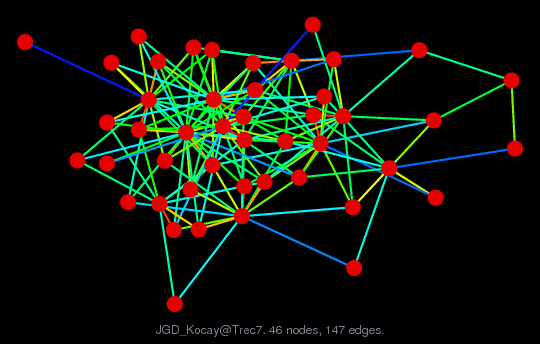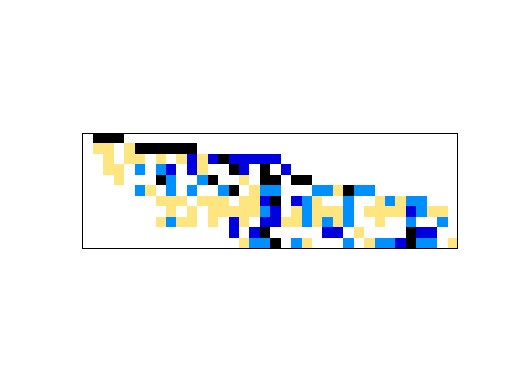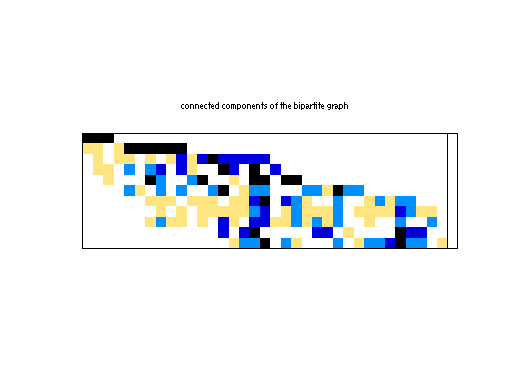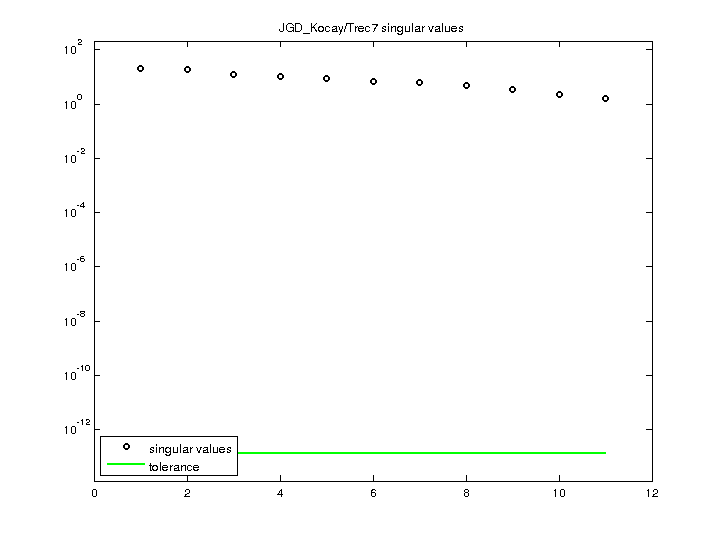Matrix: JGD_Kocay/Trec7

Description: Brute force disjoint product matrices in tree algebra on n nodes, Nicolas Thiery(bipartite graph drawing)• Matrix group: JGD_Kocay
• download as a MATLAB mat-file, file size: 2 KB. Use UFget(2141) or UFget('JGD_Kocay/Trec7') in MATLAB.

 Matrix properties number of rows 11 number of columns 36 nonzeros 147 structural full rank? yes structural rank 11 # of blocks from dmperm 1 # strongly connected comp. 2 explicit zero entries 0 nonzero pattern symmetry 0% numeric value symmetry 0% type integer structure rectangular Cholesky candidate? no positive definite? no

 author N. Thiery editor J.-G. Dumas date 2008 kind combinatorial problem 2D/3D problem? no

Notes:

```Brute force disjoint product matrices in tree algebra on n nodes, Nicolas Thiery
From Jean-Guillaume Dumas' Sparse Integer Matrix Collection,
http://ljk.imag.fr/membres/Jean-Guillaume.Dumas/simc.html

http://www.lapcs.univ-lyon1.fr/~nthiery/LinearAlgebra

Linear algebra for combinatorics

Abstract: Computations in algebraic combinatorics often boils down to
sparse linear algebra over some exact field. Such computations are
usually done in high level computer algebra systems like MuPAD or
Maple, which are reasonnably efficient when the ground field requires
symbolic computations.  However, when the ground field is, say Q or
Z/pZ, the use of external specialized libraries becomes necessary. This
document, geared toward developpers of such libraries, present a brief
overview of my needs, which seems to be fairly typical in the
community.

Filename in JGD collection: Kocay/Trec7.txt2
```

 Ordering statistics: result nnz(V) for QR, upper bound nnz(L) for LU, with COLAMD 214 nnz(R) for QR, upper bound nnz(U) for LU, with COLAMD 60

 SVD-based statistics: norm(A) 21.0361 min(svd(A)) 1.64601 cond(A) 12.78 rank(A) 11 sprank(A)-rank(A) 0 null space dimension 0 full numerical rank? yes

 singular values (MAT file): click here SVD method used: s = svd (full (R)) ; where [~,R,E] = spqr (A') with droptol of zero status: ok